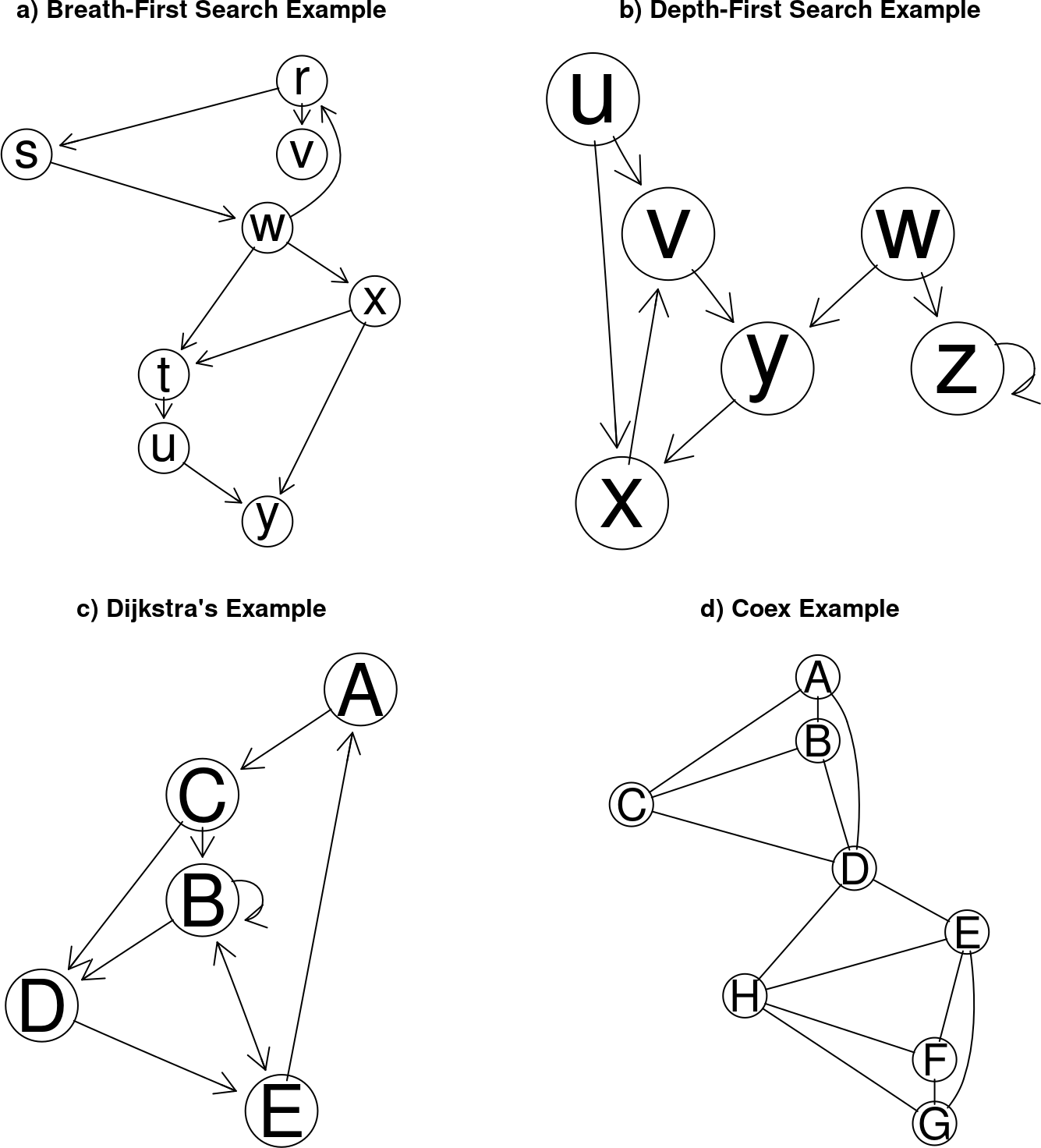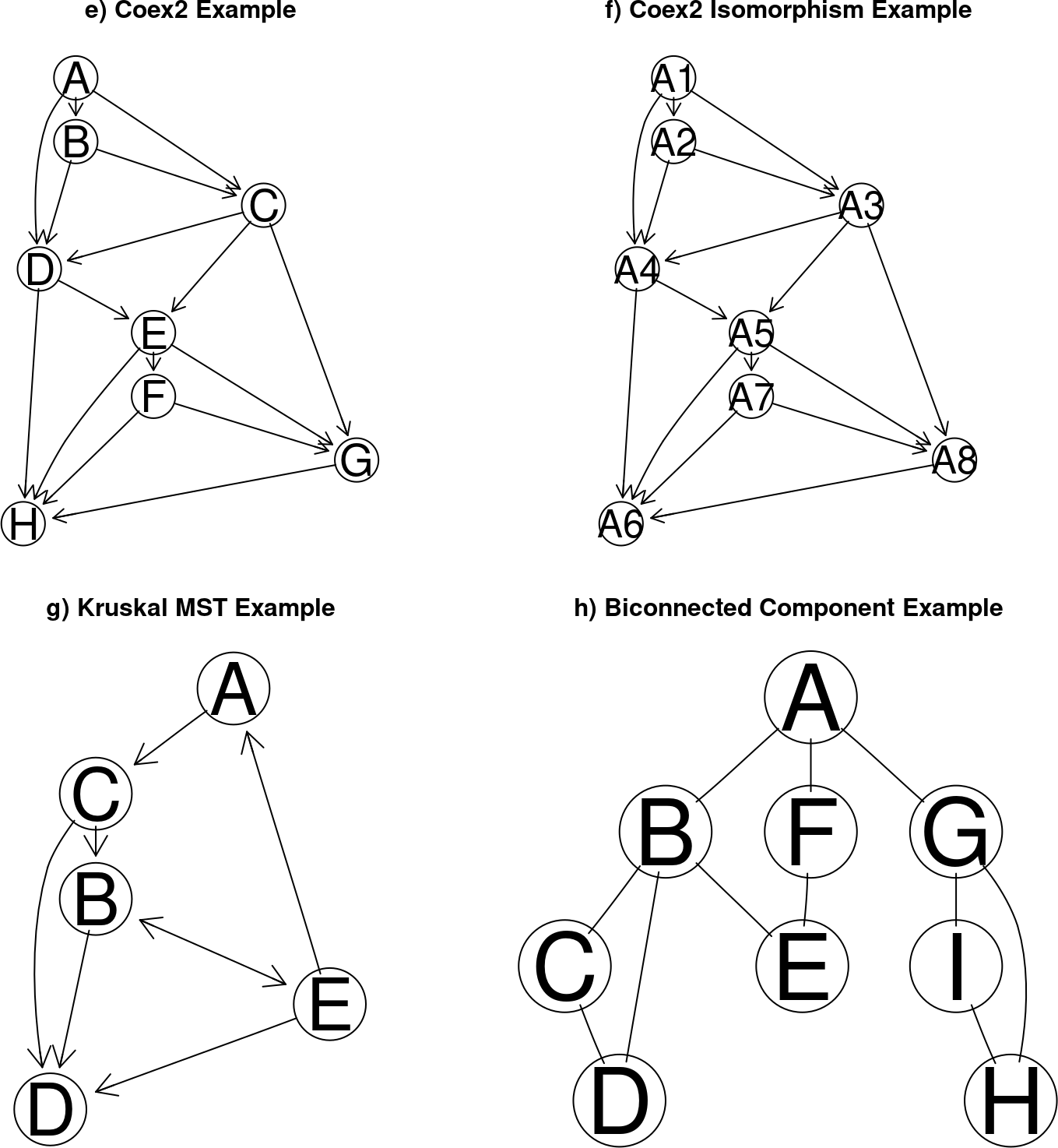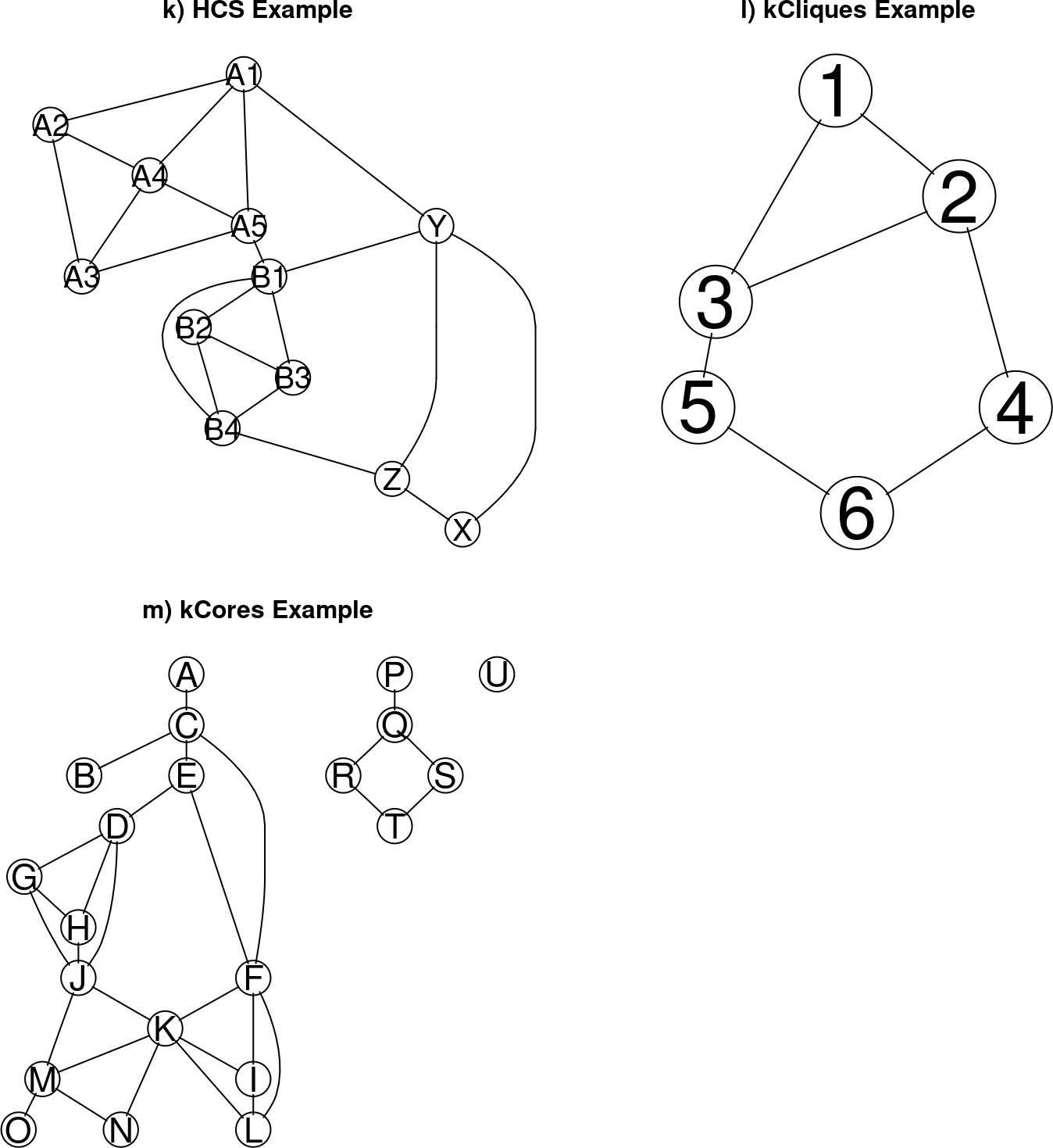# Contents

Summary. The RBGL package is primarily an interface from R to the Boost Graph Library (BGL). It includes some graph algorithms built on top of those from BGL and some algorithms independent of BGL.

# 1 Basic notations/Preliminaries

## 1.1 Basics Notations

We use the following notation:

G: a graph, represented as G = (V, E); V = v1, v2, …, vn: a set of vertices (or nodes); E = e1, e2, …, em: a set of edges with ei = [vj, vk], with vj, vk are in V; W = w1, w2, …, wm: a set of weights of the edges, i.e., wi is the weight on edge ei.

A walk is a sequence of vertices v1, v2, …, vk such that for all i, [vi, vi+1] in E. A path is a walk without repeated vertices. A cycle is a path that begins and ends at the same vertice.

A directed graph is a graph with direction assigned to its edges, therefore, [vj, vk] != [vk, vj].

A directed acyclic graph (DAG) is a directed graph with no directed cycle.

An in-degree of vertex v is the total number of edges [u, v] in E; an out-degree of v is the total number of edges [v, u] in E.

A network N is a directed graph G with:

1. a source s whose in-degree is 0,
2. a sink t whose out-degree is 0, and
3. a capacity for each edge in a network.

A flow in N assigns a value on each edge that doesn’t exceed its capacity, all the internal vertices have the same incoming flow as the outgoing flow, s has outgoing flow only, t has incoming flow only.

## 1.2 Examples in use

We are going to use the following graphs repeatedly in the examples.

``````con <- file(system.file("XML/bfsex.gxl", package="RBGL"))
bf <- fromGXL(con)
close(con)``````
``````con <- file(system.file("XML/dfsex.gxl", package="RBGL"))
df <- fromGXL(con)
close(con)``````
``````con <- file(system.file("XML/dijkex.gxl", package="RBGL"))
dijk <- fromGXL(con)
close(con)``````
``````con <- file(system.file("XML/conn.gxl", package="RBGL"))
coex <- fromGXL(con)
close(con)``````
``````con <- file(system.file("XML/conn2.gxl", package="RBGL"))
coex2 <- fromGXL(con)
close(con)``````
``````con <- file(system.file("XML/conn2iso.gxl", package="RBGL"))
coex2i <- fromGXL(con)
close(con)``````
``````con <- file(system.file("XML/kmstEx.gxl", package="RBGL"))
km <- fromGXL(con)
close(con)``````
``````con <- file(system.file("XML/biconn.gxl", package="RBGL"))
bicoex <- fromGXL(con)
close(con)``````
``````con <- file(system.file("XML/ospf.gxl", package="RBGL"))
ospf <- fromGXL(con)
close(con)``````
``````con <- file(system.file("dot/joh.gxl", package="RBGL"))
joh <- fromGXL(con)
close(con)``````
``````con <- file(system.file("XML/hcs.gxl", package="RBGL"))
hcs <- fromGXL(con)
close(con)``````
``````con <- file(system.file("XML/snacliqueex.gxl", package="RBGL"))
kclex <- fromGXL(con)
close(con)``````
``````con <- file(system.file("XML/snacoreex.gxl", package="RBGL"))
kcoex <- fromGXL(con)
close(con)``````Figure 1: The example graphs (I)Figure 2: The example graphs (II)Figure 3: The example graphs (III)